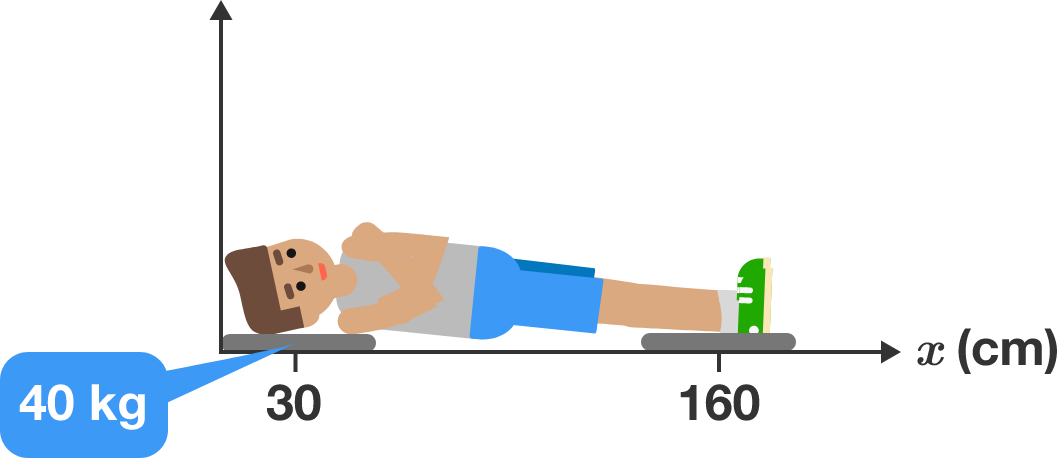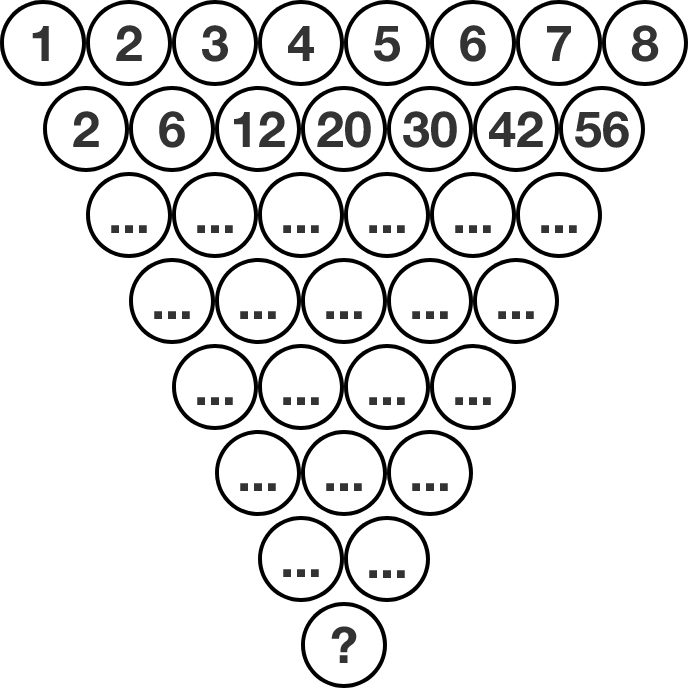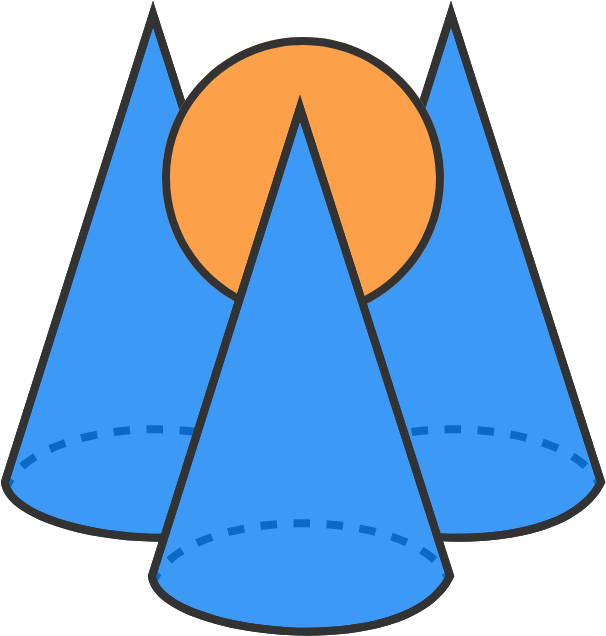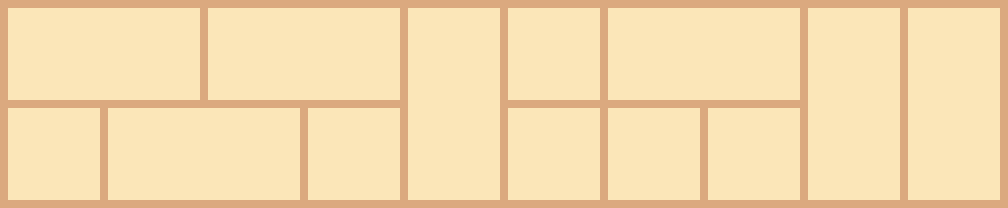# Problems of the Week

Contribute a problem

# 2018-02-26 Intermediate

Maurice is 180 cm tall. When he stands on a bathroom scale, it reads 75 kg.

Now Maurice lies down on his back, supporting his body with a bathroom scale under his neck (30 cm from the top of his head) and with another scale under his calf (160 cm from the top of his head).

The scale under Maurice's neck reads 40 kg. What is the location of Maurice's center of mass, measured from the top of his head? (Round to the nearest centimeter, if necessary.)Let $X$ and $Y$ be positive integers such that the first few digits of the fractions $\dfrac{29}{{\color{#D61F06}{70}}},\ \dfrac{{\color{#D61F06}{70}}}{{\color{#3D99F6}{169}}},\ \dfrac{{\color{#3D99F6}{169}}}{{\color{#20A900}{408}}},\ \dfrac{{\color{#20A900}{408}}}{{\color{#624F41}{X}}},\ \dfrac {{\color{#624F41}{X}}}{{\color{grey}{Y}}}$ are all equal to 0.4142. What is ${{\color{#624F41}{X}}}+{{\color{grey}{Y}}}?$

Note: The fractions are not equal.

The image shows a triangular formation of circles, where the top row has numbers 1 through 8. The circles in subsequent rows each have the product of the two numbers above it. For example, below the $2$ and $3$ is $2\times 3 = 6.$

How many trailing zeros will the number at the bottom have?Three identical cones each have a radius of 50 and a height of 120. The cones are placed such that their circular bases touch one another. Then a sphere is placed such that it rests in the space created by the three cones, as shown.

If the top of the sphere is the same height as the top of the cones, what is the radius of the sphere to three decimal places?

How many ways can a $2 \times 10$ rectangle be filled with $1 \times 1$ and $2 \times 1$ tiles?

One such possible tiling is shown below.Note: Rotations are allowed, so the $2 \times 1$ tiles can be placed either horizontally or vertically. This problem is intended to be solved with programming.

×